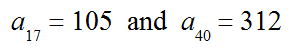# 9110 Arithmetic First Three From 2 Terms

4 Questions | Total Attempts: 65Settings• 1.
Given two terms of an arithmetic sequence below, what is the first term of this sequence?
• 2.
Given two terms of an arithmetic sequence below, what is the first term of this sequence?
• 3.
Given two terms of an arithmetic sequence below, what is the first term of this sequence?
• 4.
Given two terms of an arithmetic sequence below, what is the first term of this sequence?
• 5.
Given two terms of an arithmetic sequence below, what is the first term of this sequence?
• 6.
Given two terms of an arithmetic sequence below, what is the first term of this sequence?
• 7.
Given two terms of an arithmetic sequence below, what is the first term of this sequence?
• 8.
Given two terms of an arithmetic sequence below, what is the second term of this sequence?
• 9.
Given two terms of an arithmetic sequence below, what is the third term of this sequence?
• 10.
Given two terms of an arithmetic sequence below, what is the second term of this sequence?
• 11.
Given two terms of an arithmetic sequence below, what is the second term of this sequence?
• 12.
Given two terms of an arithmetic sequence below, what is the second term of this sequence?
• 13.
Given two terms of an arithmetic sequence below, what is the second term of this sequence?
• 14.
Given two terms of an arithmetic sequence below, what is the second term of this sequence?
• 15.
Given two terms of an arithmetic sequence below, what is the second term of this sequence?
• 16.
Given two terms of an arithmetic sequence below, what is the third term of this sequence?
• 17.
Given two terms of an arithmetic sequence below, what is the third term of this sequence??
• 18.
Given two terms of an arithmetic sequence below, what is the third term of this sequence?
• 19.
Given two terms of an arithmetic sequence below, what is the third term of this sequence?
• 20.
Given two terms of an arithmetic sequence below, what is the third term of this sequence?
• 21.
Given two terms of an arithmetic sequence below, what is the third term of this sequence?
• 22.
Given two terms of an arithmetic sequence below, what is the fifth of this sequence?
• 23.
Given two terms of an arithmetic sequence below, what is the fifth of this sequence?
• 24.
Given two terms of an arithmetic sequence below, what is the fifth of this sequence?
• 25.
Given two terms of an arithmetic sequence below, what is the fifth of this sequence?
Related Topics